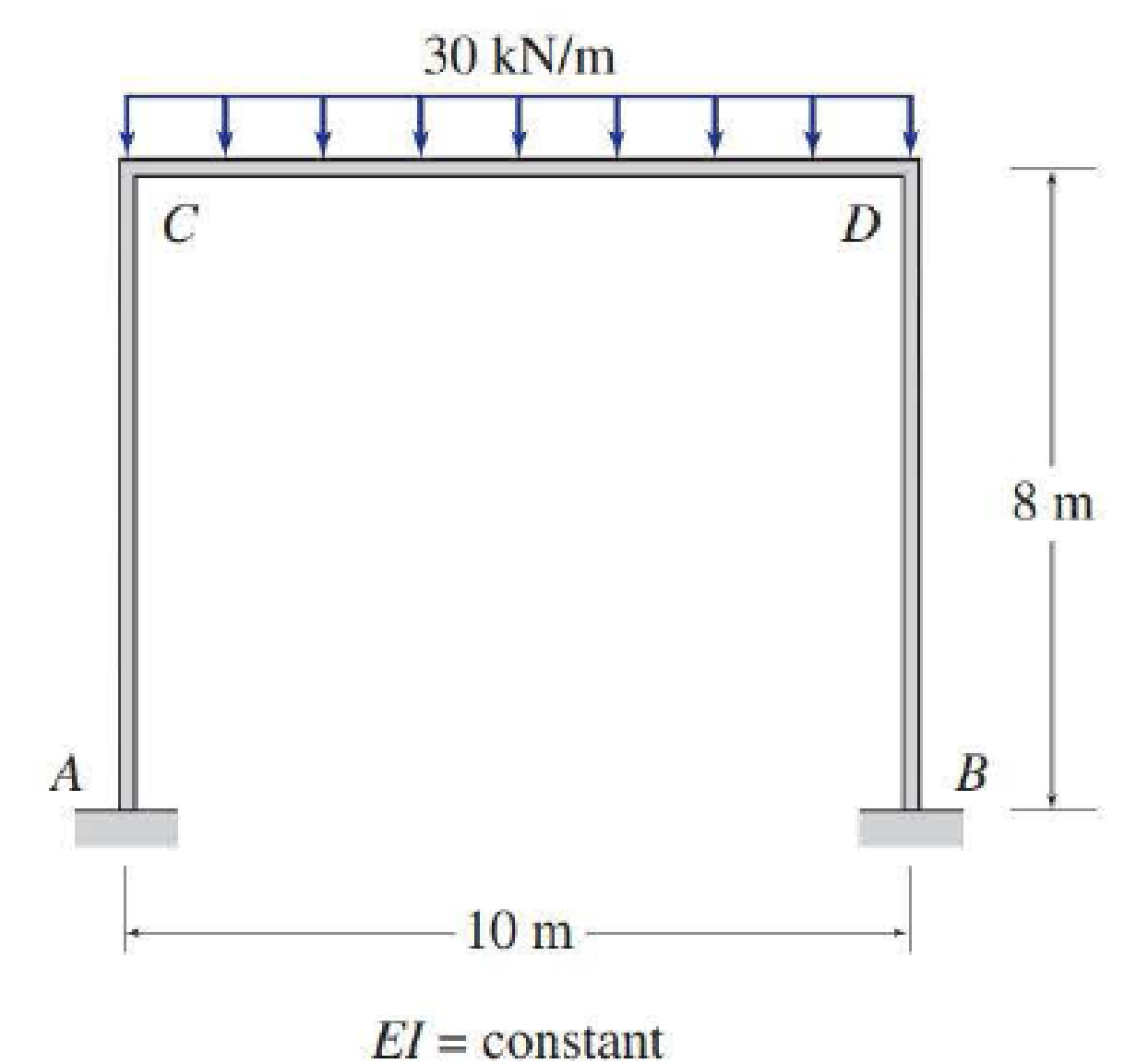# Determine the member end moments and reactions for the frames shown in Figs. P15.17–P15.20 by using the slope-deflection method. FIG. P15.20

#### Solutions

Chapter
Section
Chapter 15, Problem 20P
Textbook Problem
5 views

## Determine the member end moments and reactions for the frames shown in Figs. P15.17–P15.20 by using the slope-deflection method.FIG. P15.20

To determine

Find the member end moments and reaction for the frames.

### Explanation of Solution

Fixed end moment:

Formula to calculate the fixed moment for UDL is WL212.

Calculation:

Consider the flexural rigidity EI of the frame is constant.

Show the free body diagram of the entire frame as in Figure 1.

Refer Figure 1,

Calculate the fixed end moment for AC.

FEMAC=0

Calculate the fixed end moment for CA.

FEMCA=0

Calculate the fixed end moment for CD.

FEMCD=30×10212=250kNm

Calculate the fixed end moment for DC.

FEMDC=250kNm

Calculate the fixed end moment for DB.

FEMDB=0

Calculate the fixed end moment for BD.

FEMBD=0

Calculate the slope deflection equation for the member AC.

MAC=2EIL(2θA+θC3ψ)+FEMAC

Substitute 0 for ψ, 0 for θA, 8 m for L and 0 for FEMAC.

MAC=2EI8(2(0)+θC3(0))+0=0.25EIθC        (1)

Calculate the slope deflection equation for the member CA.

MCA=2EIL(2θC+θA3ψ)+FEMCA

Substitute 0 for ψ, 0 for θA, 8 m for L, and 0 for FEMCA.

MCA=2EI8(2θC+θA3(0))+0=0.5EIθC        (2)

Calculate the slope deflection equation for the member BD.

MBD=2EIL(2θB+θD3ψ)+FEMBD

Substitute 0 for ψ, 0 for θB, 8 m for L, and 0 for FEMBD.

MBD=2EI8(0+θD3(0))+0=0.25EIθD        (3)

Calculate the slope deflection equation for the member DB.

MDB=2EIL(2θD+θB3ψ)+FEMDB

Substitute 0 for ψ, 0 for θB, 8 m for L, and 0 for FEMDB.

MDB=2EI8(0+2θD3(0))+0=0.5EIθD        (4)

Calculate the slope deflection equation for the member CD.

MCD=2EIL(2θC+θD3ψ)+FEMCD

Substitute 0 for ψ, 10 m for L, and 250kNm for FEMCD.

MCD=2EI10(2θC+θD3(0))+250=0.4EIθC+0.2EIθD+250        (5)

Calculate the slope deflection equation for the member DC.

MDC=2EIL(2θD+θC3ψ)+FEMDC

Substitute 0 for ψ, 10 m for L, and 250kNm for FEMDC.

MDC=2EI10(2θD+θC3(0))250=0.2EIθC+0.4EIθD250        (6)

Write the equilibrium equation as below.

MCA+MCD=0

Substitute equation (2) and equation (5) in above equation.

0.5EIθC+0.4EIθC+0.2EIθD+250=00.9EIθC+0.2EIθD+250=00.9EIθC+0.2EIθD=250        (7)

Write the equilibrium equation as below.

MDB+MDC=0

Substitute equation (4) and equation (6) in above equation.

0.5EIθD+0.2EIθC+0.4EIθD250=00.9EIθD+0.2EIθC250=00.9EIθD+0.2EIθC=250        (8)

Solve the equation (7) and (8).

θC=357.14EIkNm2θD=357.14EIkNm2

Substitute 357.14EIkNm2 for θC in equation (1)

### Still sussing out bartleby?

Check out a sample textbook solution.

See a sample solution

#### The Solution to Your Study Problems

Bartleby provides explanations to thousands of textbook problems written by our experts, many with advanced degrees!

Get Started

Find more solutions based on key concepts
These dimensions concern transportation systems. Look up the dimensions of the following vehicles; give the mod...

Engineering Fundamentals: An Introduction to Engineering (MindTap Course List)

What is the difference between projecting and joining?

Principles of Information Systems (MindTap Course List)

Why do APC ferrules create less back reflection than UPC ferrules?

Network+ Guide to Networks (MindTap Course List)

The nucleus of an atom contains and

Automotive Technology: A Systems Approach (MindTap Course List)

What happens when you press a toggle key?

Enhanced Discovering Computers 2017 (Shelly Cashman Series) (MindTap Course List)

What are the Cartesian coordinates of the four points shown at A, B. C, and D?

Precision Machining Technology (MindTap Course List)

What factors are important in a DBMS software selection?

Database Systems: Design, Implementation, & Management

Why are entity integrity and referential integrity important in a database?

Database Systems: Design, Implementation, & Management

List some applications that use low-pressure piping.

Welding: Principles and Applications (MindTap Course List)

If your motherboard supports ECC DDR3 memory, can you substitute non-ECC DDR3 memory?

A+ Guide to Hardware (Standalone Book) (MindTap Course List)